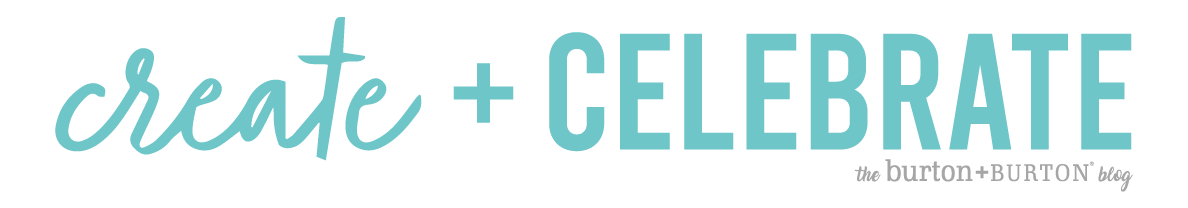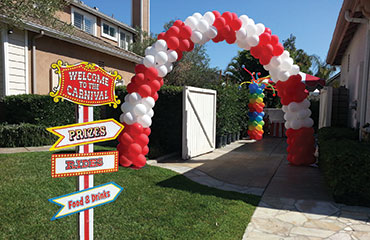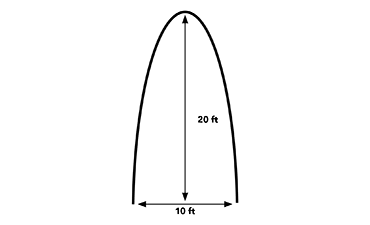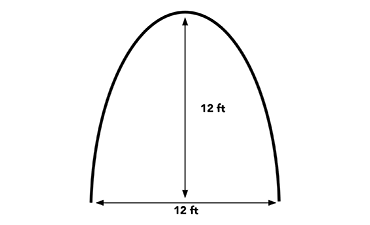Top# Calculating a Balloon Arch or GarlandClassic balloon décor will always be the bread and butter of any balloon business, but sometimes calculating a correct quote for your customer can be challenging. The ability to accurately complete a job cost form is the difference between a profitable balloon décor job and a day wasted.

The number one question our Customer Service team at burton + BURTON® receives is, “How many balloons do I need to create a balloon arch?” This question seems easy enough to answer, but many aspects come into play when calculating the number of balloons needed to make a balloon arch. What does the space look like? How are you going to size your balloons? Will you be using four or five balloon clusters to create the arch?

Customers calling a balloon decorator are usually unaware of the many variables that determine the cost of a design, so it’s important to be ready to give a quick estimate. Balloon arches range in cost based on size and materials used. With a few simple calculations, you will be able to deliver design quotes with ease. Below are examples showing several different types of balloon arches:

Tall Arches:

If an arch is taller than it is wide–for example, an arch fit for a standard door entrance–you should double the height and add the width to give the approximate total length. For example, an arch 20 feet in height and 10 feet in width will use this formula:

Step 1: Total length

2(Height) + Width = Total Length

Double the height, which is 20 feet2 to get 40 feet. Now add the width of 10 to get an approximate total length of 50 feet.

Step 2: Convert length in feet to inches

50 feet12 = 600 inches

Step 3: Total balloons used

Finally, factor in the size of your balloons and the number of balloons in each cluster used in your design. In this example, we will use 11-inch standard latex sized to 10 inches.

600 inches/10 inches = 60 clusters needed

4 cluster design: 60 clusters4 = 240 total balloons used

5 cluster design: 60 clusters5 = 300 total balloons usedWide Arches:

To calculate an arch that is wider than it is tall, like an arch over a football field, simply add the height and length to give you the approximate total length. For example, an arch that is 12 feet tall and 20 feet wide will use this formula.

Step 1: Total length

Height + Width = Length

Add the 12-foot height to the 20-foot width to give you the approximate total length of 32 feet.

Step 2: Convert length in feet to inches

32 feet12 = 384 inches

Step 3: Total balloons used

Finally, you will need to factor in the size of your balloons and the number of balloons in each cluster used in your design. In this example, we will use 11-inch standard latex sized to 10 inches.

384 inches/10 inches = 38.4 clusters needed

4 cluster design: 38.4 clusters4 = 153.6 rounded up to 154 total balloons used

5 cluster design: 38.4 clusters5 = 192 total balloons usedEqual Height and Width Arches:

If the height and width are the same or similar in measure, multiply one and a half times the height and add the width to get the approximate length. For example, an arch that is both 12 feet tall and 12 feet wide will use this formula.

Step 1: Total length

1.5(Height) + Width = Length

Multiply the height of 12 feet by 1.5 and then add the width of 12 feet to reach an approximate total length of 30 feet.

Step 2: Convert length in feet to inches

30 feet12 = 360 inches

Step 3: Total balloons used

Finally, factor in the size of your balloons and the number of balloons in each cluster used in your design. In this example, we will use 11-inch standard latex sized to 10 inches.

360 inches/10 inches = 36 clusters needed

4 cluster design: 36 clusters4 = 144 total balloons used

5 cluster design: 36 clusters5 = 180 total balloons usedTo determine how many bags of each color balloon will be needed to create the desired effect, use this simple equation for a standard spiral design:

Number of clusters/Number of colors = Number of each color balloon

Remember to make allowances to accommodate popped balloons and design errors when placing your order. When pricing your design, also factor in added perceived value when special patterns or adornments like twisted shapes or foil balloons are used.

The sky is the limit when it comes to what you can create! We can’t wait to see your designs. Make sure to tag us on Instagram @burtonplusburton for a chance to be featured!